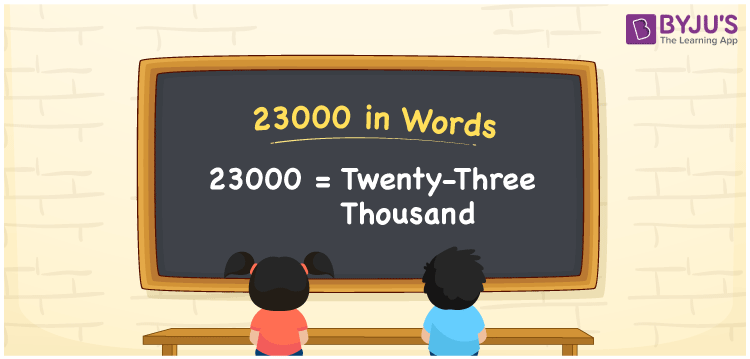# 23000 in Words

23000 in wordsis written as Twenty three Thousand. 23000 represents the count or value. The article onCounting Numberscan give you an idea about count or counting. The number 23000 is used in expressions that relate to money, distance, length and many more. In the case of Money, Rs 23000 is used as Twenty Three Thousand Rupees in cheques, discussion in banks and also while counting the money.

 23000 in words Twenty Three Thousand Twenty Three thousand in Numbers 23000

## 23000 in English Words## How to Write 23000 in Words?

We can convert 23000 to words using a place value chart. This can be done as follows. The number 23000 has 5 digits, so let’s make a chart that shows the place value up to 5 digits.

 Ten thousand Thousands Hundreds Tens Ones 2 3 0 0 0

Thus, we can write the expanded form as:

2 × Ten thousand + 3 × Thousand + 0 × Hundred + 0 × Ten + 0 × One

= 2 × 10000 + 3 × 1000 + 0 × 100 + 0 × 10 + 0 × 1

= 23000

= Twenty Three Thousand.

23000 is the natural number that is succeeded by 22999 and preceded by 23001.

23000 in words – Twenty Three thousand.

Is 23000 an odd number? – No.

Is 23000 an even number? – Yes.

Is 23000 a perfect square number? – No.

Is 23000 a perfect cube number? – No.

Is 23000 a prime number? – No.

Is 23000 a composite number? – Yes.

## Solved Example

1. Write the number 23000 in expanded form

Solution: 2 x 10000 + 3 x 1000 + 0 x 100 + 0 x 10 + 0 x 1

Or Just 2 x 10000 + 3 x 1000

We can write 23000 = 20000 + 3000 + 0 + 0 + 0

= 2 x 10000 + 8 x 1000 + 0 x 100 + 0 x 10 + 0 x 1

## Frequently Asked Questions on 23000 in words

### How to write 23000 in words?

23000 in words is written as Twenty Three Thousand.

### State True or False. 23000 is divisible by 4?

True. 23000 is divisible by 4.

### Is 23000 divisible by 10?

Yes. 23000 is divisible by 10. It is also divisible by 2, 4, 5, 10, 100, 1000.